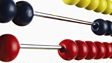A child makes a call on his phone. He has 10p to spend and his call costs him 2p. How much he has left can be calculated using subtraction 10p - 2p = 8p. Another child makes a call, he has 10p to spend and his call costs 8p. Again we calculate how much he has left using subtraction 10p - 8p = 2p. Both of these are subtraction facts of ten. Each of these subtraction calculations are shown together as the clip highlights that if we know one subtraction fact we can find another by rearranging the subtracting number and the answer.This clip is from:
The Maths Channel, Year 1 - Subtraction